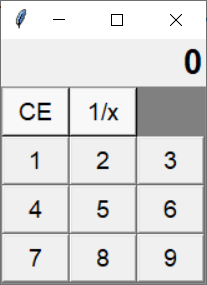TORONTO KIDS COMPUTER CLUB | Aurora Saturday 18:00 Python Homework 20.06.27.
17841

# Aurora Saturday 18:00 Python Homework 20.06.27.

## 03 Jul Aurora Saturday 18:00 Python Homework 20.06.27.

Question:
Continue to program the code we made in the class at the bottom of this page. Try to use for loop to create buttons from 1-9, so the tkinter window would display as below:Hint:
You can use “for i in range(1, 10):” or “for i in ‘123456789’:”  to build these 9 buttons, however, you should thinking of how to use math techniques to solve the row and column.

for example, buttons 1,2,3 are in row 1 and buttons 4,5,6 are in row 2. You should think that int(1/3) is 0 or 1//3 is 0,  2//3 is still 0, 3//3 is 1, 4//3 is 1, 5//3 is 1, 6//3 is 2. Then how can you make 1,2,3 all become 1, while 4,5,6 all become 2?

You should use %(finding division remainder) to solve columns, but how?

You can finish the code below:

```from tkinter import *

class Calculator:
def __init__(self, master):
self.master = master
self.master.title('Calculator')

self.f1 = Frame(self.master)
self.f1.pack(expand=YES, fill=BOTH)

self.strDisplay = StringVar()
self.strDisplay.set('0')
self.lblDisplay = Label(self.f1,
textvariable=self.strDisplay,
justify='right')
self.lblDisplay.pack(side=RIGHT)

self.f2 = Frame(self.master)
self.f2.pack(expand=YES, fill=BOTH)

self.btnCE = Button(self.f2, text='CE', bg='#f8f8f8', width=4, command=self.reset, bd=1)
self.btnCE.grid(row=0, column=0)
self.btnRecip = Button(self.f2, text='1/x', bg='#f8f8f8', width=4, command=self.recip, bd=1)
self.btnRecip.grid(row=0, column=1)
self.btnBack = Button(self.f2, text='<-', bg='#f8f8f8', width=4, command=self.back, bd=1)
self.btnBack.grid(row=0, column=2)

for i in range(1, 10):
btnNum = Button(self.f2, text=______, bg='#f8f8f8', width=4, bd=1)
btnNum.grid(row=________, column=__________)

def back(self):
pass

def reset(self):
pass

def recip(self):
pass

tk = Tk()
myCal = Calculator(tk)
tk.mainloop()```